6.12 Circles - Part 3:

6.12.1: Arcs of a circle

Two arcs of two different circles having same radii are said to be ‘congruent’ if their central angles are same.

Arc ASB = Arc CTD if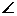AOB =CO’D

6.12.1 Theorem 1: If two arcs are congruent then their chords are equal

To prove: AB=CD

Proof:

 1. OA = O’C, OB = O’D (Radii) 2.AOB =CO’D (it is given that arcs are congruent) Hence by SAS Postulate on congruence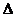AOB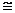CO’D Hence AB = CD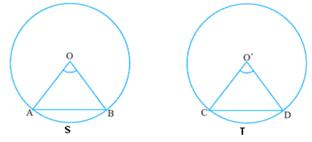6.12.1 Theorem 2: If two chords of circles having same radii are same, then their arcs are congruent.

Note: This is converse of the previous theorem. (Use SSS postulate to show thatAOB =CO’D)

6.12.1: Areas of sectors/segments of circle

 If ‘r’ is the radius of a circle, we know that : Circumference of the circle = 2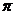r, Area of the circle =r2,                                  Whereis a constant whose approximate value we use for our calculations is 22/7 (3.1428).   Let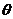(whereis in degrees) be the angle at center (COD) formed by the arc CSD. Since  3600  at  the central angle  gives us  2r as the perimeter of the circle  then  fordegree at the center, length of the arc made by it is 1. Length of the arc CSD = (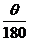) *r (unitary method) Since  3600  at  the central angle  gives usr2 as the area of the circle  then fordegree at the center, area of the sector made bydegrees:   2. Area of the sector CSDO (shaded portion in the adjoining figure) = (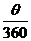) *r2 = () * (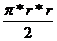)= [() *r]*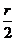= Length of the arc*(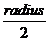) Note:radians = 1800 and x0 = (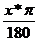) radians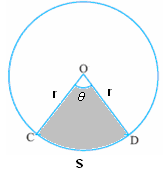LetCOD =in the adjoining figure with CD as chord We note that Area of triangle CDO =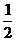*base*height =*DO*CM =*r*rsin=r2*sin(CM = rsin: Refer to section 7.1 for definition of sin of an angle)   From the figure we notice that Area of Sector CSDO = Area of triangle CDO + Area of segment CSDArea of segment  CSD  = Area of Sector CSDO - Area ofCDO = () *r2 - () r2*sin= r2 {(*) - (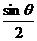)} Note: For all the above calculationsmust be in degrees.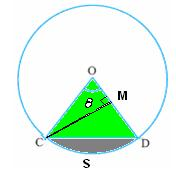6.12 Problem 1: AB and CD are respectively arcs of two concentric circles of radii 21Cm and 7Cm with center as O. IfAOB= 30,     find the area of the shaded portion

 Area of the shaded portion CABD= area of OCABDO-area of OCDO =  (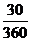) *212  - () *72 (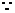212=72 *32)   =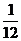*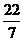*7*7*(3*3-1) =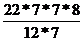=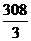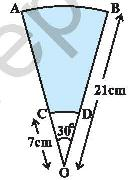6.12 Problem 2: In the figure, ABC is a quadrant of a circle of radius 14 cm and a semicircle is drawn with BC as diameter. Find the area of the shaded region

 Area of shaded region BPCQB= Area of semi circle BCQB- Area of sector  BCPB But Area of sector BCPB = Area of quarter of circle ACPB- Area ofABCArea of shaded region BPCQB =  Area of semi circle BCQB – ( Area of  quarter of circle  ACPB- Area ofABC) =  Area of semi circle BCQB – Area of quarter of circle ACPB + Area ofABC Note AC=AB=14  andBAC=90  By Pythagoras theorem BC2 =  AB2 + AC2BC(diameter) =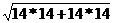=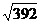.Radius  of semi circle BCQB=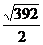Area of semi circle BCQB =*=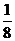**392= 154 Area of quarter of circle ACPB =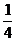142 (one fourth area of circle of radius 14cm)=**14*14=154 Area ofABC =*14*14 = 98 (Base and height of the triangle are same )Area of shaded region BPCQB= 154 -154 + 98 =98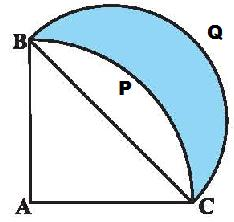6.12 Summary of learning

 No Points to remember 1 Congruency of arcs 2 Formula for length of an arc, Area of a segment